Open in App
Not now

# Class 10 RD Sharma Solutions – Chapter 15 Areas Related to Circles – Exercise 15.4 | Set 1

• Last Updated : 28 May, 2021

### Question 1. A plot is in the form of a rectangle ABCD having semi-circle on BC as shown in the figure. If AB = 60 m and BC = 28 m, find the area of the plot.

Solution: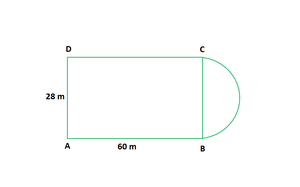Given that, ABCD is a rectangle

So, AB = CD = 60 m

And, BC = AD = 28 m

So, the radius of the semi-circle = BC/2 = 28/2 = 14 m

Now we find the area of the plot = Area of rectangle ABCD + Area of semi – circle

= (l x b) + 1/2 πr2

= (60 x 28) + 1/2 (22/7)(14)2

= 1680 + 308

= 1988 cm2

Hence, the area of the plot is 1988 cm2

### Question 2. A play ground has the shape of a rectangle, with two semi-circles on its smaller sides as diameters, added to its outside. If the sides of the rectangle are 36 m and 24.5 m, find the area of the playground. (Take π = 22/7).

Solution: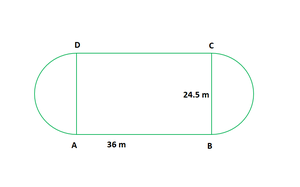Given that,

Length of rectangle = 36 m

Breadth of rectangle = 24.5 m

So, the radius of the semi-circle = breadth/2 = 24.5/2 = 12.25 m

Now we find the area of the playground = Area of the rectangle + 2 x area of semi-circles

= l x b + 2 x 1/2 (πr2)

= (36 x 24.5) + (22/7) x (12.25)2

= 882 + 471.625 = 1353.625

Hence, the area of the playground is 1353.625 m2

### Question 3. Find the area of the circle in which a square of area 64 cm2 is inscribed. (Use π = 3.14)

Solution: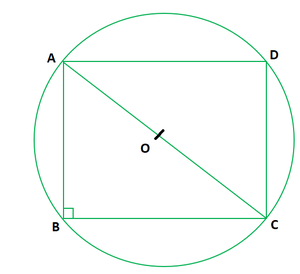Given that,

Area of square inscribed the circle = 64 cm2

Side2 = 64

So, Side = 8 cm

So, AB = BC = CD = DA = 8 cm

Now In triangle ABC,

By using Pythagoras theorem, we get

AC2 = AB2 + BC2

AC2 = 82 + 82

AC2 = 64 + 64 = 128

AC = √128 = 8√2 cm

So, the angle B = 90o and AC being the diameter of the circle

and the radius is AC/2 = 8√2/2 = 4√2 cm

Now we find the area of the circle = πr2 = 3.14(4√2)2

= 100.48 cm2

Hence, the area of the circle is 100.48 cm2

### Question 4. A rectangular piece is 20 m long and 15 m wide. From its four corners, quadrants of radii 3.5 m have been cut. Find the area of the remaining part.

Solution: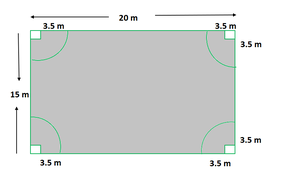Given,

Length of the rectangle = 20 m

Breadth of the rectangle = 15 m

Now we find the area of the remaining part = (Area of the rectangle) – 4 x( Area of one quadrant)

= (l x b) – 4 x (1/4 x πr2)

= (l x b) – πr2

= (20 x 15) – (22/7)(3.5)2

= 300 – 38.5

= 261.5 m2

Hence, the area of the remaining part is 261.5 m2

### Question 5. In the figure, PQRS is a square of side 4 cm. Find the area of the shaded square.

Solution: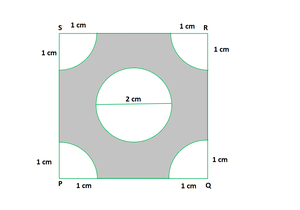From the above figure we know that, each quadrant is a sector of 90o in

a circle of 1 cm radius. In other words its 1/4th of a circle.

So, its area = 1/4 x πr2

= 1/4 x (22/7)(1)2 = 22/28 cm2

And, the area of the square = side2

It is given that the side = 4 cm

So, 42 = 16 cm2

The area of the circle = πr2 = π(1)2 = 22/7 cm2

Now we find the area of the shaded region = area of the square – area of the circle – 4 x area of a quadrant

= 16 – 22/7 – (4 x 22/28)

= 16 – 22/7 – 22/7 = 16 – 44/7

= 68/7 cm2

Hence, the area of the shaded region is 68/7 cm2

### Question 6. Four cows are tethered at four corners of a square plot of side 50 m, so that they just cannot reach one another. What area will be left ungrazed? (See figure)

Solution: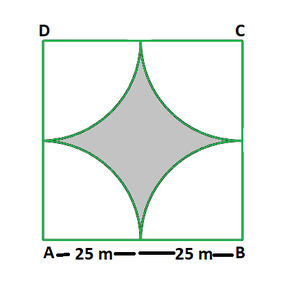Given,

Side of square plot = 50 m

Now we find the area of plot left un-grazed = Area of the plot – 4 x (area of a quadrant)

= Side2 – 4 x (1/4 x πr2)

= (50)2 – 22/7 x (25)2

= 2500 – 1964.28

= 535.72 m2

Hence, the area of plot left un-grazed is 535.72 m2

### Question 7. A cow is tied with a rope of length 14 m at the corner of a rectangular field of dimensions 20 m x 16 m, find the area of the field in which the cow can graze.

Solution: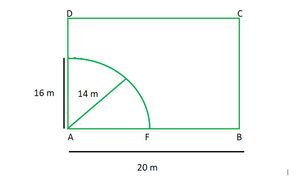The dotted portion is the area over which the cow can graze.

So, from the above figure, the shaded area is the area of a quadrant

of a circle of radius equal to the length of the rope.

According to the question it is given that the length of the rope is 14m

So, the radius of the circle is 14 cm

Now we find the required area = 1/4 x πr2

= 1/4 x 22/7 x (14)2

= 154 cm2

Hence, the area of the field in which the cow can graze is 154 cm2

### Question 8. A calf is tied with a rope of length 6 m at the corner of a square grassy lawn of side 20 m. If the length of the rope is increased by 5.5 m, find the increase in the area of the grassy lawn in which the calf can graze.

Solution: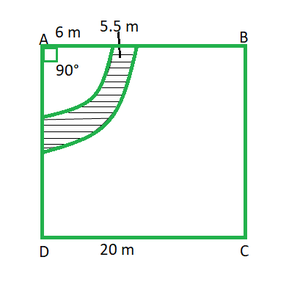Given,

The initial length of the rope = 6 m

Then the rope is said to be increased by 5.5m

So, the increased length of the rope = (6 + 5.5) = 11.5 m

We know that, the corner of the lawn is a quadrant of a circle.

Now we find the required area = 1/4 x π(11.5)2 – 1/4 x π(5.5)2

= 1/4 x 22/7 (11.52 – 62)

= 1/4 x 22/7 (132.25 – 36)

= 1/4 x 22/7 x 96.25

= 75.625 cm2

Hence, the increase in area of the grassy lawn in which the calf can graze is 75.625 cm2

### Question 9. A square water tank has its side equal to 40 m. There are four semi-circular grassy plots all round it. Find the cost of turfing the plot at Rs.1.25 per square meter (Take π = 3.14).

Solution: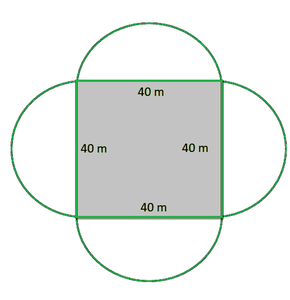Given,

Side of the square tank = 40 m

And, the diameter of the semi-circular grassy plot = side of the square tank = 40 m

So, the radius of the grassy plot = 40/2 = 20 m

Now we find the area of the four semi-circular grassy plots = 4 x 1/2 πr2

= 4 x 1/2 (3.14)(20)2

= 2512 m2

Rate of turfing the plot = Rs 1.25 per m2

So, the cost for 2512 m2 = (1.25 x 2512) = Rs 3140

Hence, the area of the four semi-circular grassy plots is 2512 m2

and the cost of turfing the plot at 1.25 per square meter is Rs 3140.

### Question 10. A rectangular park is 100 m by 50 m. It is surrounded by semi-circular flower beds all round. Find the cost of levelling the semi-circular flower beds at 60 paise per square meter. (Use π = 3.14).

Solution: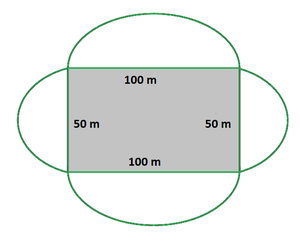Given,

Length of the park = 100 m and the breadth of the park = 50 m

The radius of the semi-circular flower beds = half of the corresponding side of the rectangular park

So, the radius of the bigger flower bed = 100/2 = 50 m

and radius of the smaller flower bed = 50/2 = 25 m

Now we find the total area of the flower beds = 2[Area of bigger flower bed + Area of smaller flower bed]

= 2[1/2 π(50)2 + 1/2 π(25)2]

= π[(50)2 + (25)2]

= 3.14 x [2500 + 625]

= 9812.5 m2

So, rate of levelling flower bed = 60 paise per m2

The total cost of levelling = 9812.5 x 60 = 588750 paise

= Rs 5887.50

Hence, the cost of levelling the semi-circular flower beds at 60 paise per square meter is Rs 5887.50

### Question 11. The inside perimeter of a running track (shown in the figure) is 400 m. The length of each of the straight portion is 90 m and the ends are semi-circles. If the track is everywhere 14 m wide, find the area of the track. Also, find the length of the outer running track.

Solution: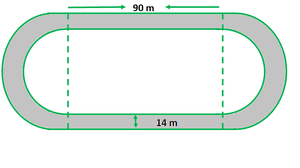Let us considered the radius of the inner semi-circle = r

And that of the outer semi-circle = R

According to the question

The length of the straight portion = 90 m

Width of the track = 14 m

The inner perimeter of the track = 400 m

So, the Inner perimeter of the track = BF + FRG + GC + CQB = 400

90 + πr + 90 + πr = 400

2 πr + 180 = 400

2 x 22/7 x r = 220

r = 35 m

So, the radius of the outer semi-circle = 35 + 14 = 49 m

Now we find the area of the track = 2[Area of the rectangle AEFB + Area of semi-circle APD – Area of semi-circle BQC]

= 2[90 x 14 + 1/2 x 22/7 x 492 – 1/2 x 22/7 x 352]

= 2[1260 + 11 x 7 x 49 – 11 x 5 x 35]

= 2 [1260 + 3773 – 1925]

= 3 x 3108

= 6216 m2

So, the length of outer running track = AE + APD + DH + HSE

= 90 + πR + 90 + πR

= 180 + 2 πR

= 180 + 2 x 22/7 x 49

= 180 + 308

= 488 m

Hence, the area of the track is 6216 m2 and the length of the outer running track is 488 m.

### Question 12. Find the area of the figure, in square cm, correct to one place of decimal. (Take π = 22/7).

Solution: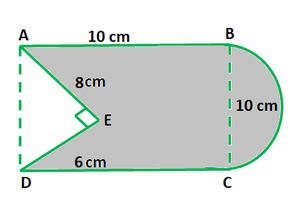The radius of the semi-circle = 10/2 = 5 cm

From the above figure,

The area of figure = Area of square + Area of semi-circle – Area of triangle AED

= 10 x 10 + 1/2 πr2 – 1/2 x 6 x 8

= 100 + 1/2 (22/7)(5)2 – 24

= (700 + 275 – 168)/7

= (807)/7

= 115.3 cm2

Hence, the area of the figure, in square cm is 115.3 cm2

### Question 13. In the figure, from a rectangular region ABCD with AB = 20 cm, a right triangle AED with AE = 9 cm and DE = 12 cm, is cut off. On the other end, taking BC as diameter, a semicircle is added on outside the region. Find the area of the shaded region. (Use π = 22/7).

Solution: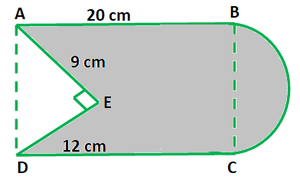Given,

Length of the rectangle ABCD = 20 cm

AE = 9 cm and DE = 12 cm

Now In triangle AED,

By using Pythagoras theorem, we get

AD = √(AE2 + ED2) = √(92 + 122)

= √(81 + 144)

= √(225) = 15 cm

So, we know that

The area of the rectangle = 20 x 15 = 300 cm2

And, the area of the triangle AED = 1/2 x 12 x 9 = 54 cm2

So, the radius of the semi-circle = 15/2 = 7.5 cm

Area of semi-circle = 1/2 π(15/2)2 = 1/2 x 3.14 x 7.52 = 88.31 cm2

Now we find the area of the shaded region = Area of the rectangle ABCD + Area of semi-circle – Area of triangle AED

= 300 + 88.31 – 54

= 334.31 cm2

Hence, the area of the shaded region is 334.31 cm2

### Question 14. From each of the two opposite corners of a square of side 8 cm, a quadrant of a circle of radius 1.4 cm is cut. Another circle of diameter 4.2 cm is also cut from the centre as shown in the figure. Find the area of the remaining (shaded) portion of the square. (Use π = 22/7).

Solution: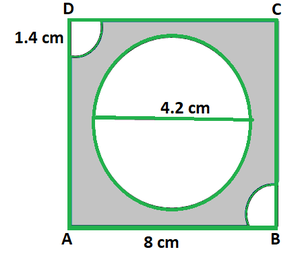Given,

Side of the square = 8 cm

Radius of circle = 4.2 cm

Now we find the area of the shaded potion = Area of square – Area of circle – 2 x Area of the quadrant

= side2 – πr2 – 2 x 1/2 πr2

= 82 – π(4.2)2 – 2 x 1/2 π(1.4)2

= 64 – 22/7(4.2 x 4.2) – 22/7(1.4 x 1.4)

= 64 – 388.08/7 – 21.56/7

= 5.48 cm2

Hence, the area of the shaded potion is 5.48 cm2

### Question 15. In the figure, ABCD is a rectangle with AB = 14 cm and BC = 7 cm. Taking DC, BC, and AD as diameters, three semi¬circles are drawn as shown in the figure. Find the area of the shaded region.

Solution: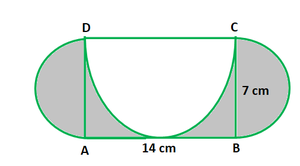Given that,

ABCD is a rectangle with AB = 14 cm and BC = 7 cm

From the above figure, we find

The area of shaded region = Area of rectangle ABCD + 2 x area of semi-circle with AD and BC as diameters – area of the semi-circle with DC as diameter

= 14 x 7 + 2 x 1/2 π(7/2)2 – 1/2 π(7)2

= 98 + 22/7 x (7/2)2 – 1/2 (22/7)(7)2

= 98 + 77/2 – 77

= 59.5 cm2

Hence, the area of the shaded region is 59.5 cm2

### (ii) the length of the boundary of the shaded region.

Solution: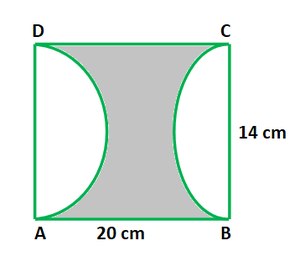Given that,

Length of the rectangle = AB = 20 cm

Breadth of the rectangle = BC = 14 cm

(i) Now we find the area of the shaded region = Area of rectangle – 2 x Area of the semi-circle

= l x b – 2 x 1/2 πr2

= 20 x 14 – (22/7) x 72

= 280 – 154

= 126 cm2

(ii) Now we find the length of the boundary of the shaded region = 2 x AB + 2 x circumference of a semi-circle

= 2 x 20 + 2 x πr

= 40 + 2 x (22/7) x 7

= 40 + 44

= 84 cm

Hence, the area of the shaded region is 126 cm2 and the length of the boundary of the shaded region is 84 cm

### (ii) the perimeter of the part ABEF.

Solution: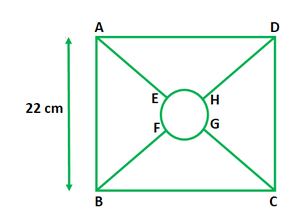Given that,

Side of the square = 22 cm = AB

Let us considered the radius of the centre part be r cm.

So, the area of the circle = 1/5 x area of the square

πr2 = 1/5 x 222

22/7 x r2 = (22 x 22)/ 5

So, the radius(r) of the circle is = 154/5 = 5.55 cm

(i) Circumference of central part = 2πr = 2(22/7)(5.55) = 34.88 cm

(ii) Let O be the centre of the central part.

Then, its clear that O is also the centre of the square as well.

Now In triangle ABC,

By using Pythagoras theorem

AC2 = AB2 + BC2 = 222 + 222 = 2 x 222

AC = 22√2

AO = 1/2 AC = 1/2 (22√2) = 11√2 cm    [Since diagonals of a square bisect each other]

And,

AE = BF = OA – OE = 11√2 – 5.55 = 15.51 – 5.55 = 9.96 cm

EF = 1/4 (Circumference of the circle) = 2πr/4

= 1/2 x 22/7 x 5.55 = 8.72 cm

Now we find the perimeter of the part ABEF = AB + AE + EF + BF

= 22 + 2 x 9.96 + 8.72

= 50.64 cm

Hence, the Circumference of central part is 34.88 cm and

the perimeter of the part ABEF is 50.64 cm

My Personal Notes arrow_drop_up